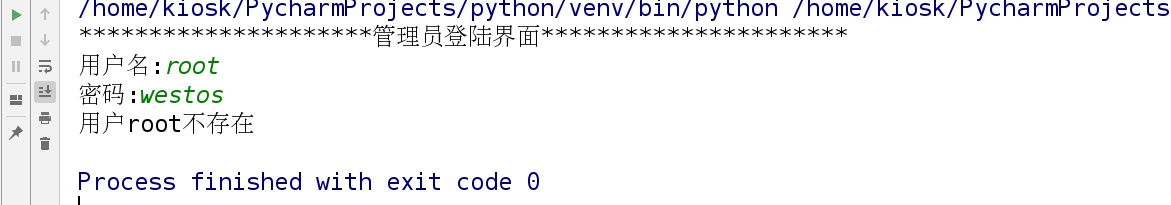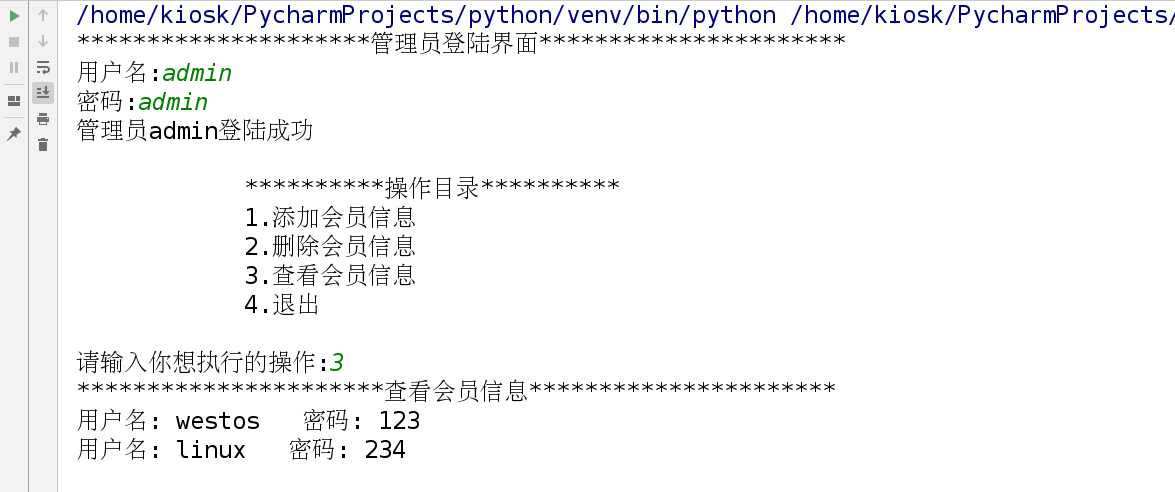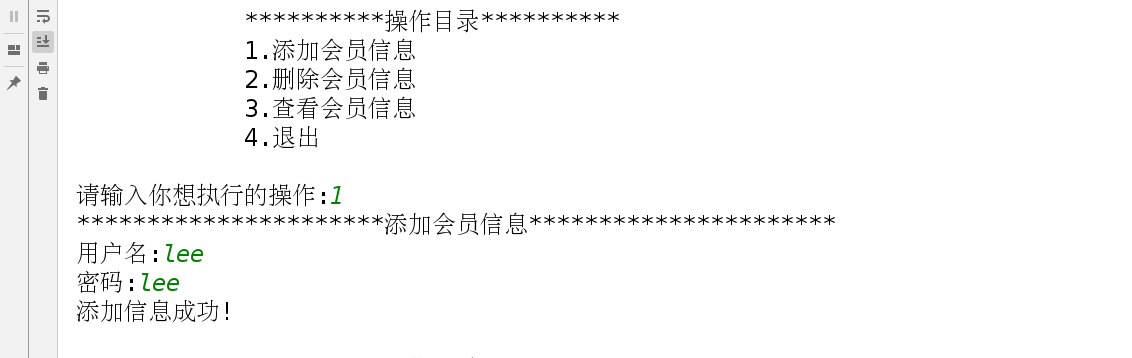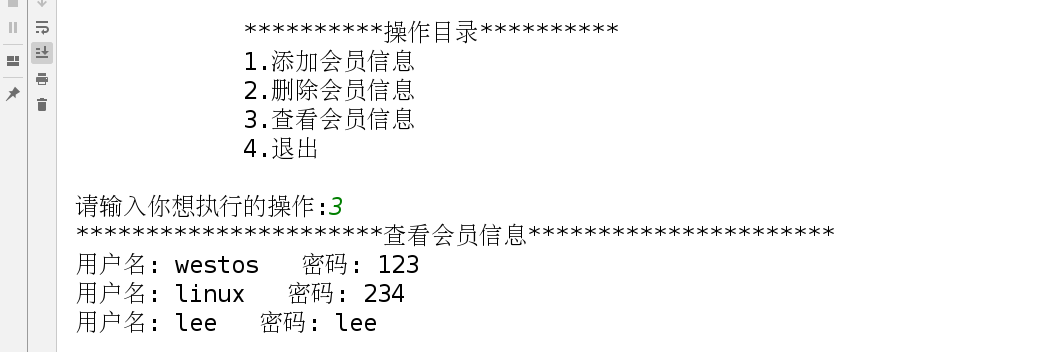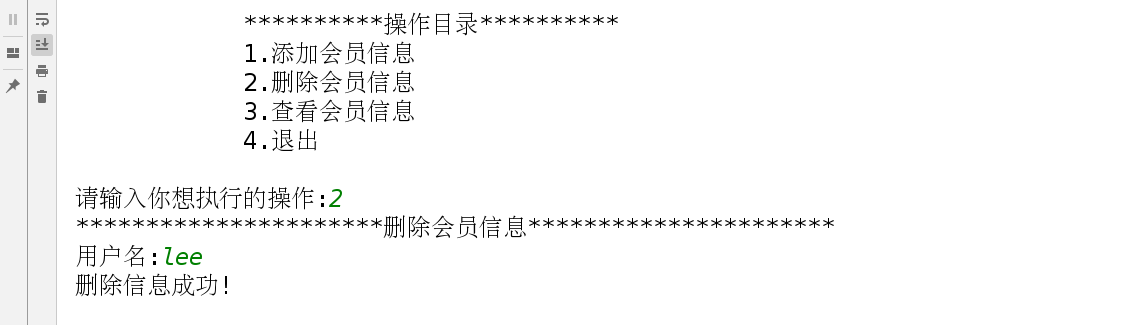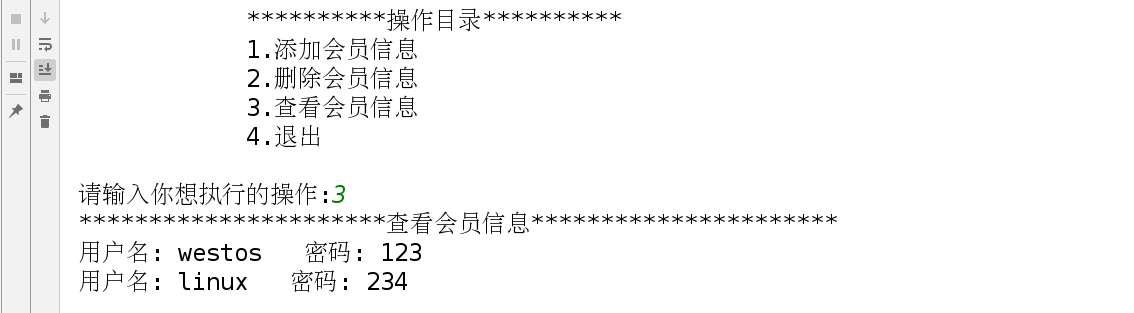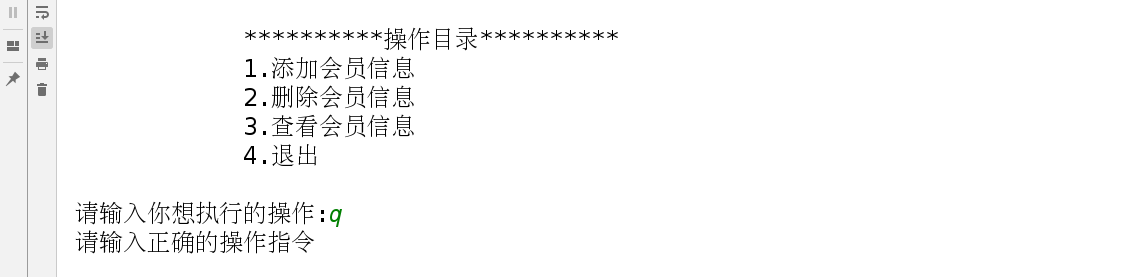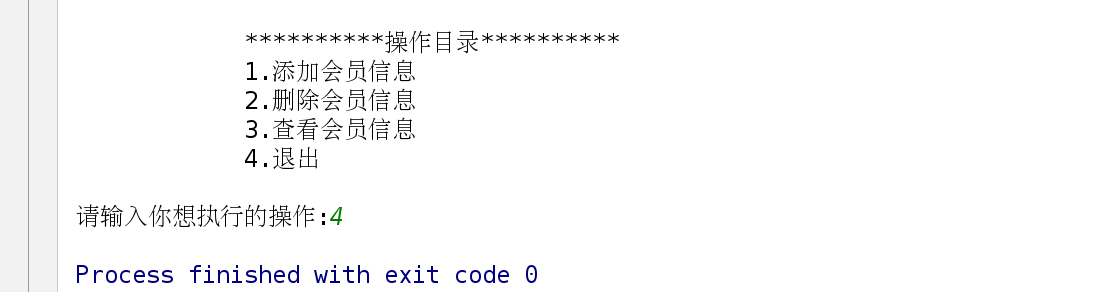# Python之列表（综合练习）

#### 列表的综合练习

1.判断季节

用户输入月份,判断这个月是哪个季节

3，4，5月----春季  6，7，8----夏季   9，10，11---秋季  12，1，2----冬季


# 接收用户输入的月份
month = int(input('month:'))

# 定义列表
spring = [3,4,5]
summer = [6,7,8]
autom = [9,10,11]
winter = [12,1,2]

# 判断输入的月份属于哪个季节
# 列表的特性：成员操作符
if month in spring:
print('%s月是春天' %(month))
elif month in summer:
print('%s月是夏天' %(month))
elif month in autom:
print('%s月是秋天' %(month))
elif month in winter:
print('%s月是冬天' % (month))
else:
print('请输入正确的月份')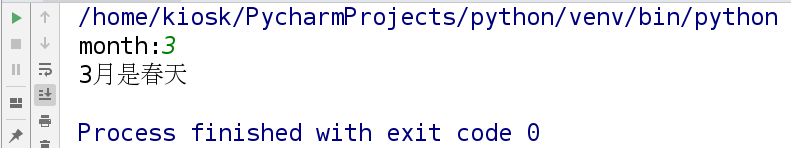2.按要求重组列表元素

假定有下面的列表:
names = ['fentiao','fendai','fensi','apple']
输出结果为:'I have fentiao, fendai, fensi and apple.'


# 定义列表
names = ['fentiao','fendai','fensi','apple']

# + 和 join 都表示连接,(join可以指定分隔符连接);
# 列表的 索引 和 切片 都相当于 分离
print('I have ' + ','.join(names[:3]) + ' and ' + (names))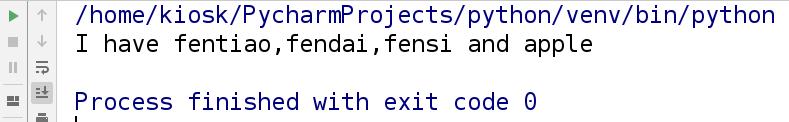3.编写小学生算术能力测试系统

设计一个程序，用来实现帮助小学生进行百以内的算术练习，它具有以



import random

# 定义用来记录总的答题数目和回答正确的数目
count = 0
right = 0

# 因为题目要求：提供10道题目(因为题目要求：提供10道题目)
while count <= 10:
# 创建列表，用来记录加减乘除四大运算符
op = ['+', '-', '*', '/']
# 随机生成op列表中的字符
s = random.choice(op)
# 随机生成0-100以内的数字
a = random.randint(0,100)
# 除数不能为0
b = random.randint(1,100)
print('%d %s %d = ' %(a,s,b))
# 默认输入的为字符串类型
question = input('请输入您的答案:(q退出)')
# 判断随机生成的运算符，并计算正确结果
if s == '+':
result = a + b
elif s == '-':
result = a - b
elif s == '*':
result = a * b
else:
result = a / b

#  判断用户输入的结果是否正确,str表示强制转换为字符串类型
if question == str(result):
print('回答正确')
right += 1
count += 1
elif question == 'q':
break
else:
print('回答错误')
count+=1
# 计算正确率
if count == 0:
percent = 0
else:
percent = right / count

print('测试结束,共回答%d道题,回答正确个数为%d,正确率为%.2f%%' %(count,right,percent*100))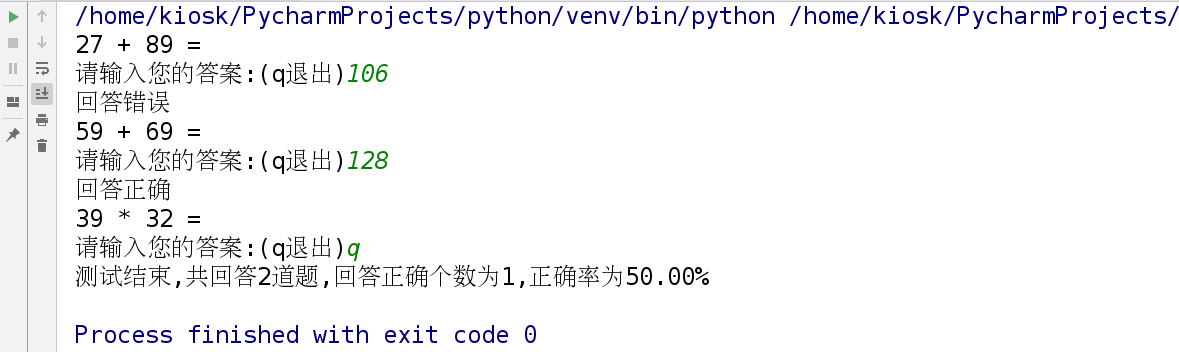4.编写用户登陆系统

1.系统里面有多个用户，用户的信息目前保存在列表里面
users = ['root','westos']
passwd = ['123','456']
2.用户登陆(判断用户登陆是否成功）
1).判断用户是否存在
2).如果存在
1).判断用户密码是否正确
如果正确，登陆成功，推出循环
如果密码不正确，重新登陆，总共有三次机会登陆
3).如果用户不存在
重新登陆，总共有三次机会


# 定义列表，用来记录用户名和密码
users = ['root','westos']
passwds = ['123','456']
# 定义尝试登陆的次数
trycount = 0

# 判断尝试登陆次数是否超过3次
while trycount < 3:
# 接收用户输入的用户名和密码
inuser = input("用户名:")
inpasswd = input("密码:")

trycount+=1
# 判断用户是否存在
if inuser in users:
# 先找出用户对应的索引值
index = users.index(inuser)
# 找出密码列表中对应的索引值的密码
passwd = passwds[index]
# 判断输入的密码是否正确
if inpasswd == passwd:
print("%s登陆成功" % (inuser))
break
else:
print("%s登陆失败: 密码错误!" % (inuser))
else:
print("用户%s不存在" % (inuser))
else:
print("已经超过三次机会")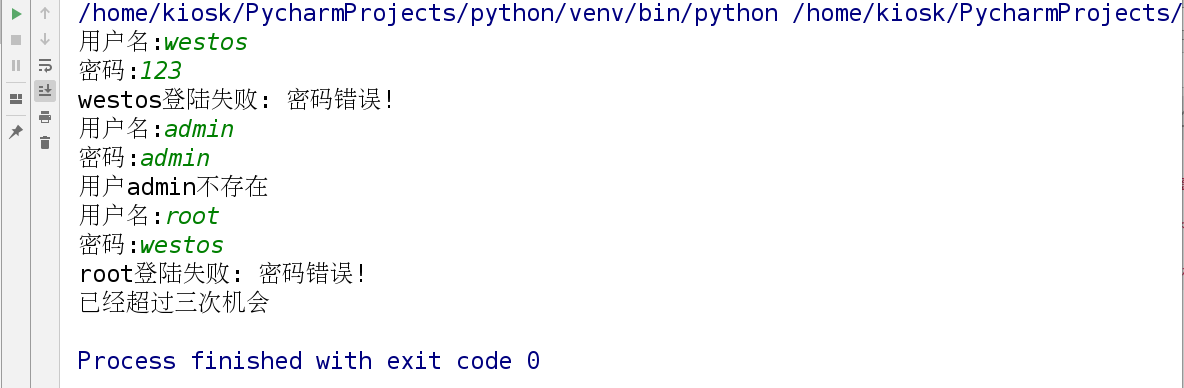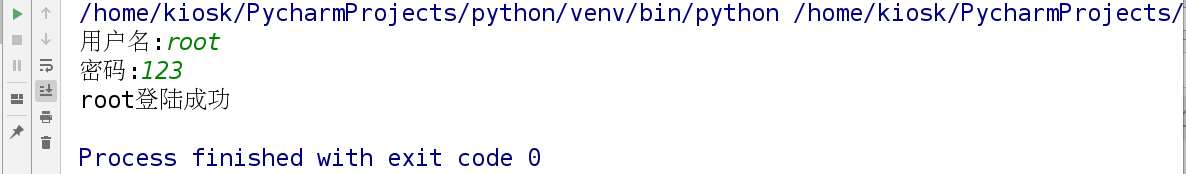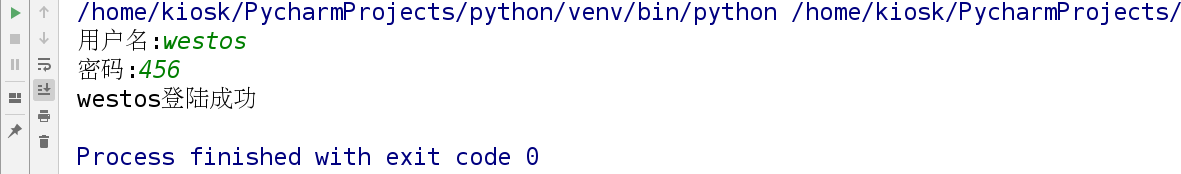5.模拟栈的工作原理

栈的工作原理
入栈
出栈
查看栈顶元素
栈的长度
栈是否为空



# 定义一个空列表,用来表示栈
stack = []

# 定义操作选项的变量
info = """
栈操作
1.入栈
2.出栈
3.栈顶元素
4.栈的长度
5.栈是否为空
q.退出
"""
# 无限循环
while True:
# 输出操作选项信息
print(info)
choice = input('请输入选择:')
if choice == '1':
print('入栈'.center(50,'*'))
# 接收入栈元素
item = input('入栈元素:')
# append:添加元素到列表中
stack.append(item)
print('元素%s入栈成功' % item)
elif choice == '2':
if len(stack) == 0:
print('栈为空,无法出栈')
else:
print('出栈'.center(50, '*'))
# pop:删除列表中的最后一个元素
item = stack.pop()
print('%s元素出栈成功' % item)
elif choice == '3':
# len:列表长度
if len(stack) == 0:
print('栈为空,无栈顶元素')
else:
print('栈顶元素为%s' % stack[-1])
elif choice == '4':
print('栈的长度为%s' % len(stack))
elif choice == '5':
if len(stack) == 0:
print('栈为空')
else:
print('栈不为空')
elif choice == 'q':
break
else:
print('请输入正确的选择')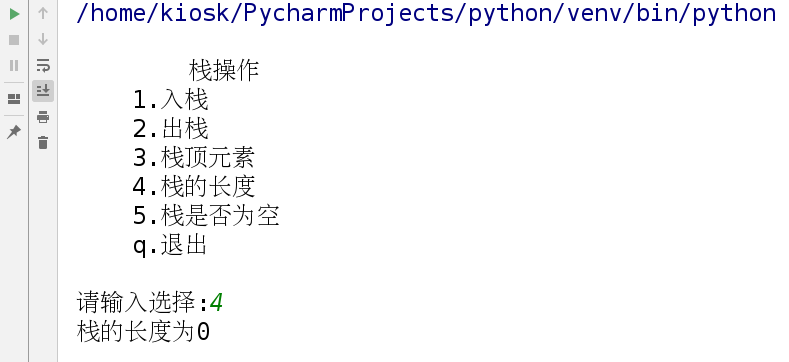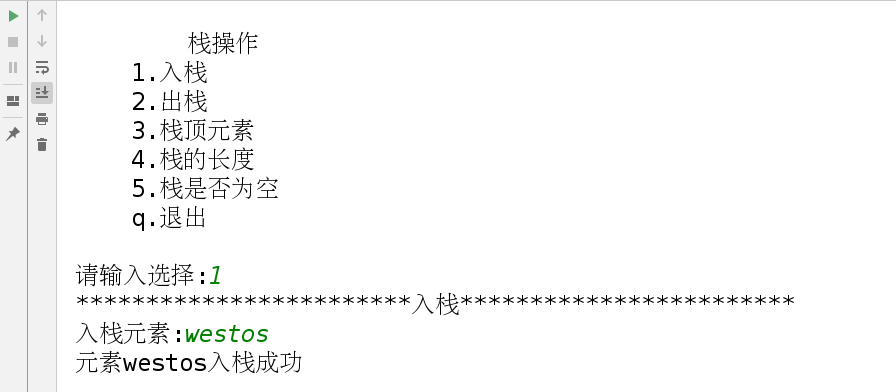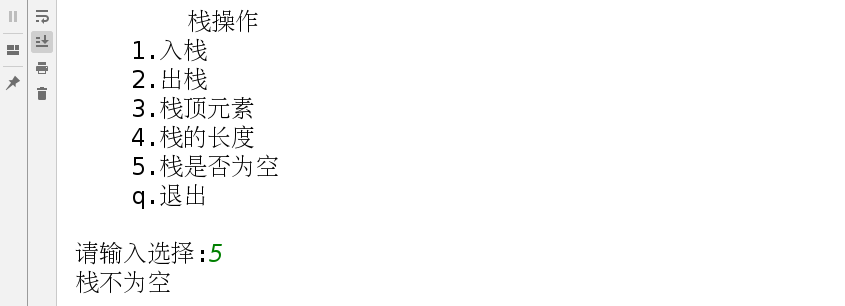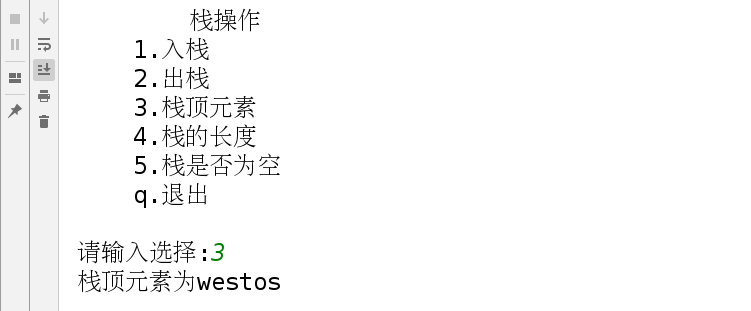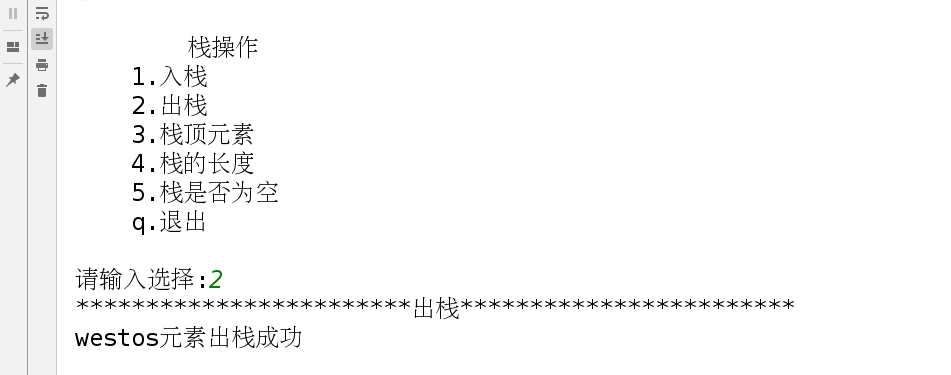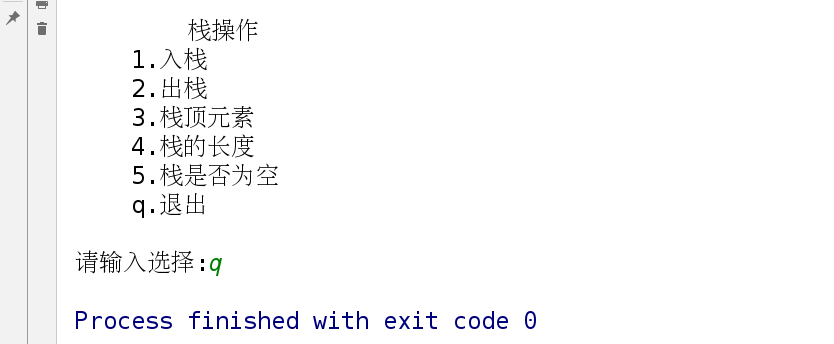6.后台管理员管理前台会员信息系统

后台管理员管理前台会员信息系统:

1. 后台管理员只有一个用户: admin, 密码: admin
2. 当管理员登陆成功后， 可以管理前台会员信息.
3. 会员信息管理包含:
添加会员信息
删除会员信息
查看会员信息
退出

- 添加用户:
1). 判断用户是否存在?
2).  如果存在， 报错；
3).  如果不存在，添加用户名和密码分别到列表中;

- 删除用户
1). 判断用户名是否存在
2). 如果存在，删除；
3). 如果不存在， 报错；


print('管理员登陆界面'.center(50,'*'))

# 初始会员信息
users = ['westos','linux']
passwds = ['123','234']

# 接收用户输入的用户名和密码
inuser = input("用户名:")
inpasswd = input("密码:")

if inuser == 'admin':
if inpasswd == 'admin':
print("管理员%s登陆成功" % (inuser))
while True:
print("""
**********操作目录**********
1.添加会员信息
2.删除会员信息
3.查看会员信息
4.退出
""")
option = input('请输入你想执行的操作:')
if option == '1':
print('添加会员信息'.center(50,'*'))
adduser = input('用户名:')
addpasswd = input('密码:')
if adduser in users:
print('添加失败，该会员信息已经存在!')
else:
users.append(adduser)
passwds.append(addpasswd)
print('添加信息成功!')
elif option == '2':
print('删除会员信息'.center(50,'*'))
deluser = input('用户名:')
if deluser not in users:
print('删除失败，该会员信息不存在!')
else:
# 找出想删除的用户对应的索引值
delindex = users.index(deluser)
# 删除用户,remove表示删除列表中的元素
users.remove(deluser)
# 按照索引值删除密码,pop也表示删除列表中的元素,区别在于,它可以按索引值来删除
passwds.pop(delindex)
print('删除信息成功!')
elif option == '3':
print('查看会员信息'.center(50,'*'))
# 记录users列表的长度（即列表中元素的个数）
count = len(users)
for i in range(0,count):
print('用户名: %s   密码: %s' %(users[i],passwds[i]))
elif option == '4':
exit()
else:
print('请输入正确的操作指令')
else:
print("%s登陆失败: 密码错误!" % (inuser))
else:
print("用户%s不存在" % (inuser))